# Statistics Quick Reference Pro

## A must have app for all Statistics students.

4.42 EDUCATION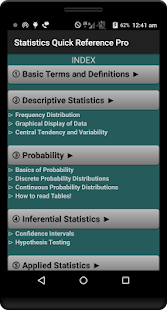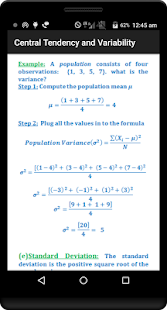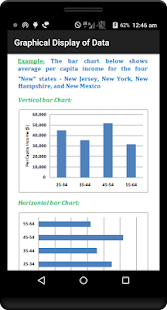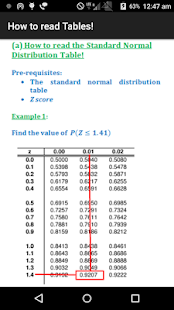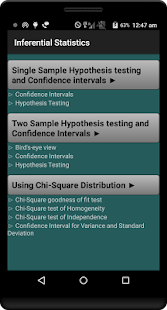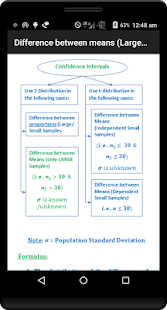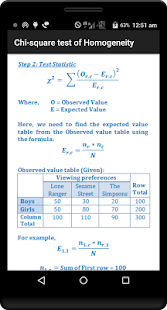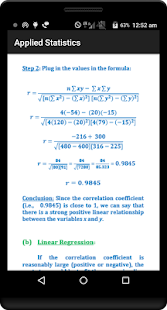This app was developed by a qualified Statistics tutor. A must have app for all Statistics students. Almost all the topics that you will need to get through your statistics course are explained in detail and simple manner.

Benefits of PRO version:

In addition to the topic covered in the free app, you will get:

- Two Sample Hypothesis Tests (Difference between proportions, Difference between means - large and small samples) explained in detail

- Two Sample Hypothesis Tests (Difference between proportions, Difference between means - large and small samples) explained in detail

- Using Chi-Square Distribution (Goodness of fit test, Test of homogeneity, Test for Independence, Confidence intervals for Standard deviation & Variance, use of chi-square distribution tables)

- No Ads

Statistics Quick Reference Pro was designed by a qualified statistics Instructor. Each of the concepts was explained in detail, followed by an example for better understanding.

The topics in the free version includes:

1)Basic Terms and Definitions

2)Descriptive Statistics
-> Frequency Distribution
-> Frequency
-> Relative Frequency
-> Cumulative Frequency
-> Cumulative Relative Frequency
-> Graphical Display of data
-> Dot Plots
-> Bar Charts
-> Histograms
-> Stem-and-leaf plots
-> Pie Charts
-> Measures of Central Tendency and Variability
-> The Mean
-> The Median
-> The Mode
-> Variance and Standard Deviation
-> The Range
-> The coefficient of Variation

3)Probability
-> Basics of Probability
-> Definitions
-> Classical Definition of Probability
-> Conditional Probability
-> Laws of Probability
-> Rules of Probability
-> Discrete Probability Distributions
-> Expected Value of Discrete Random Variable
-> Variance and Standard Deviation of Discrete Random Variable
-> Continuous Probability Distributions
-> The normal Distribution
-> The Standard Normal Distribution
-> t-Distribution
-> How to read Tables
-> The Standard Normal Distribution Table
-> t-Distribution Table

4)Inferential Statistics
-> General Guidelines (Confidence Intervals and Hypothesis Tests)
-> Large samples, Small Samples, Minimum Sample size required
-> Hypothesis Tests (Examples)
-> Confidence Intervals (Examples)

5) Applied Statistics
-> Least Squares Linear Regression(Example computing Regression equation)
-> Linear Correlation (Example computing Correlation Coefficient)

### About this version (2.2)

- Bug Fixes - Content Update - Improved UI - Compatibility with 7.X

### Details and Recent Ratings

Size: 2 MB
Version: 2.2 by Nuzedd
Updated: 18 January 2017 (1617 days ago)
Released: 05 July 2012
Installations: more than 10 000
5 Stars: 61
4 Stars: 9
3 Stars: 7
2 Stars: 2
1 Star: 5

### Similar apps

Android Game Categories
Android App Categories